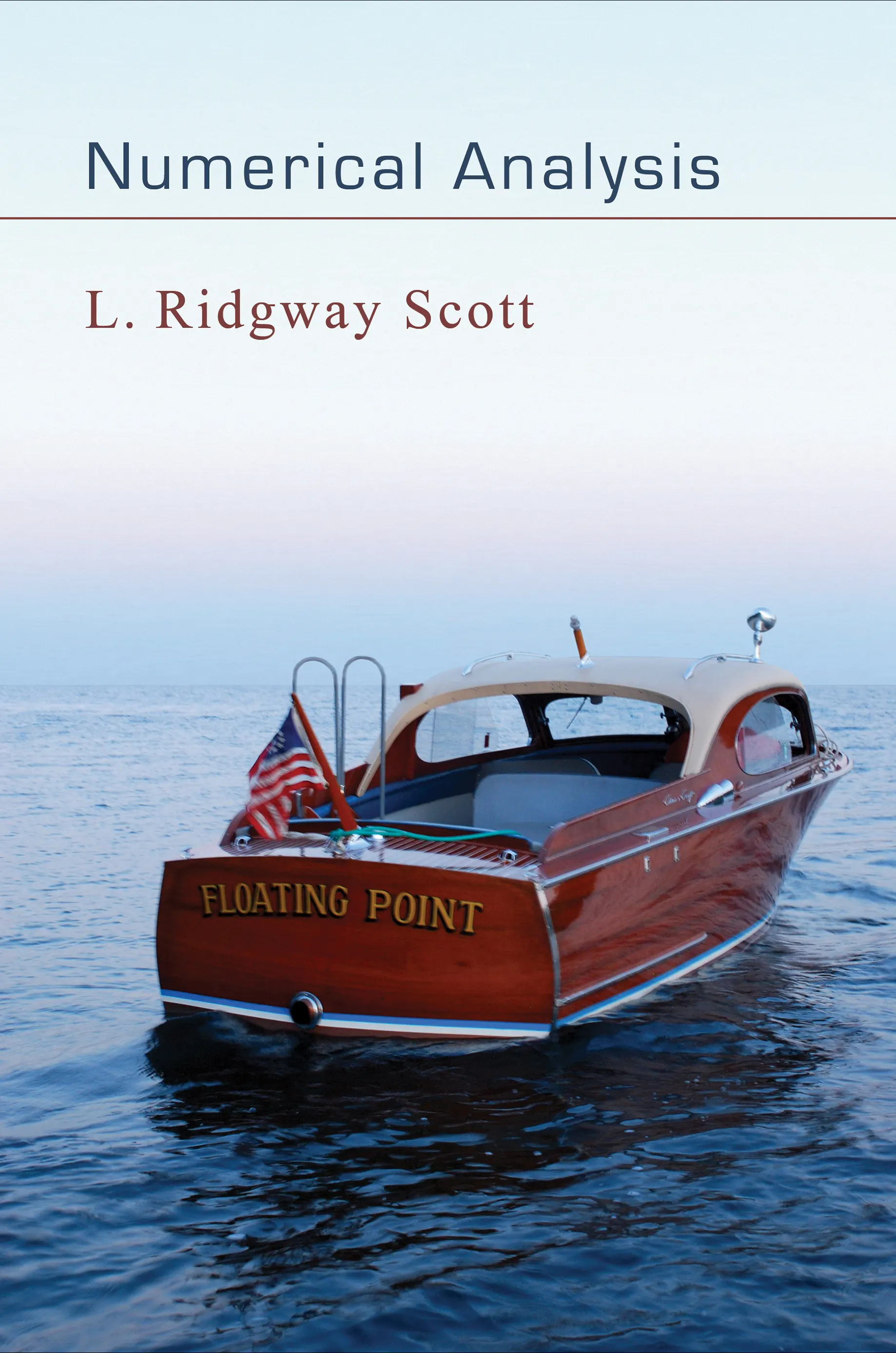#### Numerical Analysis

Larkin Ridgway Scott

30% off with code PUP30

Audiobooks and ebooks purchased from this site must be accessed on the Princeton University Press app.

# Numerical Analysis## ebook

30% off with code PUP30

Sale Price:
\$69.97/£58.80
Price:
\$99.95/£84.00
ISBN:
Published:
Apr 18, 2011
2011
Illus:
33 line illus. 11 tables.

Computational science is fundamentally changing how technological questions are addressed. The design of aircraft, automobiles, and even racing sailboats is now done by computational simulation. The mathematical foundation of this new approach is numerical analysis, which studies algorithms for computing expressions defined with real numbers. Emphasizing the theory behind the computation, this book provides a rigorous and self-contained introduction to numerical analysis and presents the advanced mathematics that underpin industrial software, including complete details that are missing from most textbooks.

Using an inquiry-based learning approach, Numerical Analysis is written in a narrative style, provides historical background, and includes many of the proofs and technical details in exercises. Students will be able to go beyond an elementary understanding of numerical simulation and develop deep insights into the foundations of the subject. They will no longer have to accept the mathematical gaps that exist in current textbooks. For example, both necessary and sufficient conditions for convergence of basic iterative methods are covered, and proofs are given in full generality, not just based on special cases.

The book is accessible to undergraduate mathematics majors as well as computational scientists wanting to learn the foundations of the subject.

• Presents the mathematical foundations of numerical analysis

• Explains the mathematical details behind simulation software

• Introduces many advanced concepts in modern analysis

• Self-contained and mathematically rigorous

• Contains problems and solutions in each chapter

• Excellent follow-up course to Principles of Mathematical Analysis by Rudin